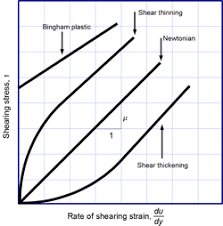## How to Calculate and Solve for Viscosity | CeramicsThe image above represents viscosity.

To compute for viscosity, three essential parameters are needed and these parameters are Force applied (F), Area (A) and Derivation Ratio of Velocity to Distance of Fluid Flow (dv/dy).

The formula for calculating viscosity:

η = F/A / dv/dy

Where:

η = Viscosity
F = Force Applied
A = Area
dv/dy = Derivation Ratio of Velocity to Distance of Fluid Flow

Let’s solve an example;
Find the viscosity when the force applied is 21, area is 14 and derivation ratio of velocity to distance of fluid flow is 19.

This implies that;

F = Force Applied = 21
A = Area = 14
dv/dy = Derivation Ratio of Velocity to Distance of Fluid Flow = 19

η = F/A / dv/dy
η = (21/14) / (19)
η = (1.5) / (19)
η = 0.0789

Therefore, the viscosity is 0.0789 Pa s.

Calculating Force Applied when the Viscosity, the Area and the Derivation ratio of velocity to distance of fluid flow is Given.

F = (η x dv/dy) A

Where;

F = Force Applied
η = Viscosity
A = Area
dv/dy = Derivation Ratio of Velocity to Distance of Fluid Flow

Let’s solve an example;
Find the force applied when the viscosity is 20, the area is 30 and the derivation is 8.

This implies that;

η = Viscosity = 20
A = Area = 30
dv/dy = Derivation Ratio of Velocity to Distance of Fluid Flow = 8

F = (η x dv/dy) A
F = (20 x 8) 30
F = (160) 30
F = 4800

Therefore, the force applied is 4800.